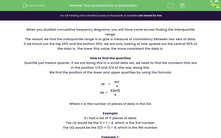# Find Quartiles from a Distribution

In this worksheet, students will find the quartiles and interquartile range from a small data set.Key stage:  KS 4

Year:  GCSE

GCSE Subjects:   Maths

GCSE Boards:   AQA, Eduqas, Pearson Edexcel, OCR,

Curriculum topic:   Statistics

Curriculum subtopic:   Statistics Analysing Data

Difficulty level:#### Worksheet Overview

When you studied cumulative frequency diagrams, you will have come across finding the interquartile range.

The reason we find the interquartile range is to give a measure of consistency between two sets of data.

If we knock out the top 25% and the bottom 25%, we are only looking at how spread out the central 50% of the data is. The lower this value, the more consistent the data is.

How to find the quartiles

Quartile just means quarter. If we are doing this in a small data set, we need to find the numbers that are in the position 1/4 and 3/4 of the way along this.

We find the position of the lower and upper quartiles by using the formula:

LQ =
 n+1 4
UQ =
 3 (n+1) 4

Where n is the number of pieces of data in the list.

Example:

If I had a list of 11 pieces of data:

The LQ would be the 11 + 1 ÷ 4, which is the 3rd number.

The UQ would be the 3(11 + 1) ÷ 4, which is the 9th number.

Example 1 :

Finding the IQR

Find the interquartile range of the data set:

21, 23, 20, 27, 25, 24, 30, 21, 22, 28, 30

Step 1: Order the data

20, 21, 21, 22, 23, 24, 25, 27, 28, 30, 30

Step 2: Find the position of the quartiles

I have 11 pieces of data, this means that the LQ is the 3rd number and the UQ is the 9th.

Step 3: Find the value of the LQ and UQ

LQ = 3rd number = 21

UQ = 9th number = 28

Step 4: Find the IQR

IQR = UQ - LQ

28 - 21 = 7

Example 2:

Finding the IQR from a frequency table

This frequency table shows the results of a survey of the number of people in a car passing a  particular point:

.

 People in a car 1 2 3 4 5 6 Frequency 45 198 121 76 52 13

Find the IQR for this data.

Step 1: Find the total frequency for this problem

We just add up all the frequencies for this. This gives us 505 cars.

Step 2: Find the position of the quartiles

Using the formula above, the LQ is the 126.5th number and the UQ is the 379.5th number.

Step 3: Find the values of the quartiles

This is a bit complicated.

If we wrote the first column out as a list, we would write 45 numbers out - have we gone past 126.5th yet?  No.

If we then write out the second column as a list, we would have 45 + 198 = 243 numbers  - have we gone past 126.5th yet?  Yes!

My LQ must be in the second column, so it must be 2.

If you do the same for the UQ, you find that UQ = 4.

Step 4: Find the IQR

IQR = UQ - LQ

4 - 2 = 2

Let's have a go at some questions now.

### What is EdPlace?

We're your National Curriculum aligned online education content provider helping each child succeed in English, maths and science from year 1 to GCSE. With an EdPlace account you’ll be able to track and measure progress, helping each child achieve their best. We build confidence and attainment by personalising each child’s learning at a level that suits them.

Get started